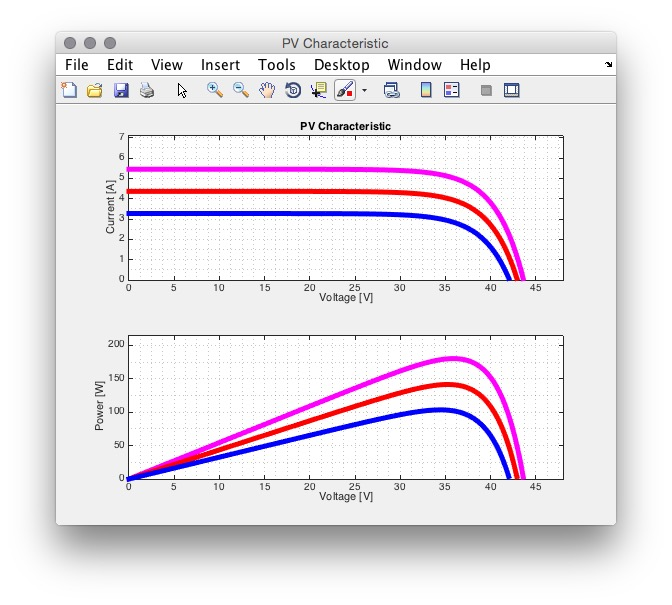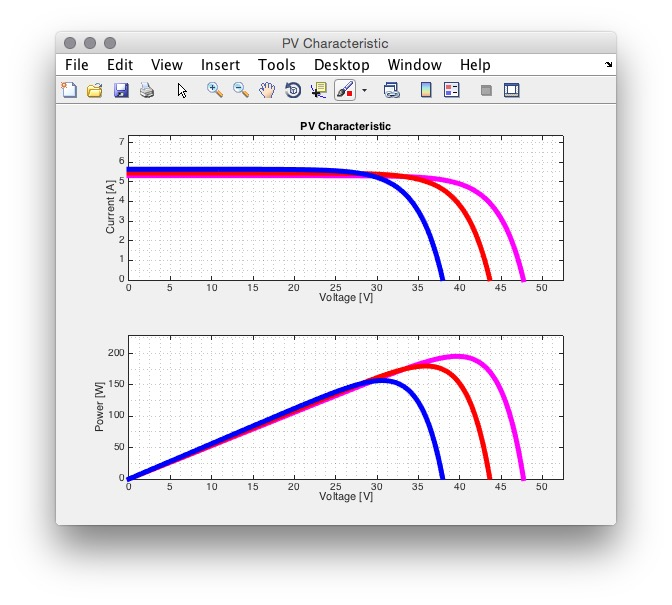## MATLAB code to create the characteristic of a PV Panel

Photovoltaic (PV) panels are not constant voltage, current or power sources, but their output depend on the temperature or irradiance levels as well as on their connected load. This is why the power electronics converters connected on the output of the panel do not operate at a particular point, but are actively taking various measurements in order to determine which is the Maximum Power Point (MPP). The implemented algorithms can perform electrical measurements (i.e. voltage and current of the module) or environmental measurements (i.e. irradiance and temperature). The system designer needs to know the PV characteristic in order to specify the current and voltage limits of the power converter and how this will vary depending on the environmental conditions. PV suppliers always provide a PV characteristic for a nominal temperature of 25C and various irradiance levels (typically 1000W/m2, 800W/m2 and 600W/m2). They also provide temperature coefficient factors, which describe the effect of the temperature value to power output. However, since the photovoltaic phenomenon is accurately mathematically described, based on those equations, the PV characteristic can be derived for any irradiance or temperature value. The "Khouzam model" provides equations to produce such a characteristic based on parameters that are available by the PV manufacturers through their datasheet. So, a MATLAB program was created in order to implement the Khouzam model. The figure below shows the output characteristic of a MODULTEQ MTS180M-24V 180W panel for three different irradiance levels, namely 1000W/m2 (magenta), 800W/m2 (red) and 600W/m2 (blue). The effect of the irradiance level is clearly shown. The maximum PV output power decreases as the irradiance level decreases. What is more, the maximum (short circuit) current level deviation is more significant than the one of the maximum (open circuit) voltage.A second figure shows the effect of the temperature on the PV panel output. The irradiance level is constant at 1000W/m2 but the PV panel temperature is 0C (magenta), 25C (red) and 60C (blue). This figure shows that when the temperature of the panel rises, the output power decreases. That is why cooling tubes that run on the back of the panels exist on certain installations. As the temperature rises the open circuit voltage decreases, whereas the short circuit current is not affected.The full project of the Khouzam equations on MATLAB can be found on my Github page.